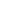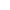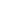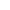# Module 07: Comparison of 1-, 2-, and 3-Parameter IRT Models

5 (1 vote)

• Learner - Free!

This module discusses the 1-, 2-, and 3-parameter logistic item response theory models. Mathematical formulas are given for each model, and comparisons among the three models are made. Figures are included to illustrate the effects of changing the a, b, or c parameter, and a single data set is used to illustrate the effects of estimating parameter values (as opposed to the true parameter values) and to compare parameter estimates achieved though applying the different models. The estimation procedure itself is discussed briefly. Discussions of model assumptions, such as dimensionality and local independence, can be found in many of the annotated references (e.g., Hambleton, 1988).

Keywords: dichotomous data, dimensionality, estimation, item response theory, IRT, local independence, model assumptions, one-parameter model, parameter estimation, two-parameter model, three-parameter model, 1PL, 2PL, 3PL

#### Key:CompleteFailedAvailableLocked
Module 7: Comparison of 1-, 2-, and 3-Parameter IRT Models